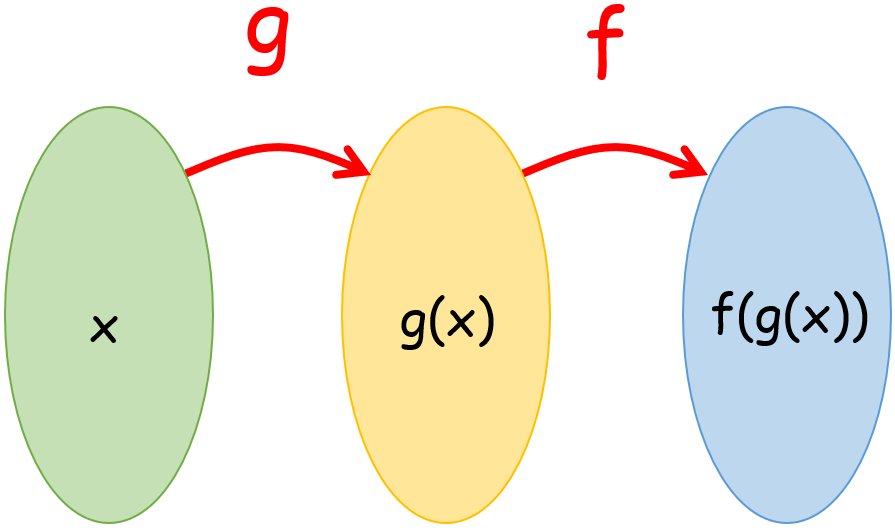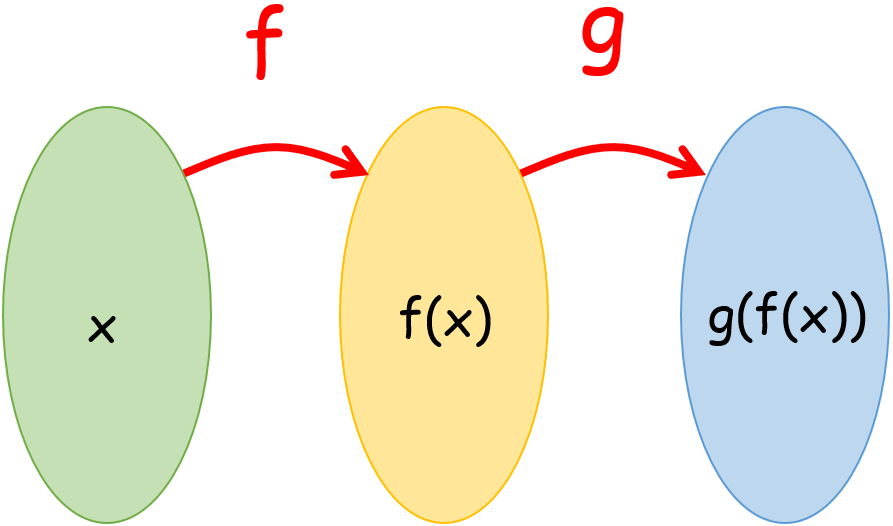## How to Solve Composite Functions

### Composition of Functions

Composing functions means combining two or more functions to make a single function.

### What is the Composition of Functions?

When $$g(x)$$ acts first, the combination of functions $$f(x)$$ and $$g(x)$$ is shown by $$f(g(x))$$ or $$(f ∘ g)(x)$$. It brings together two or more functions to make a new function. When functions are put together, the output of the function inside the parentheses becomes the input of the function outside the parentheses. So,

For $$f(g(x))$$, $$g(x)$$ is the input of $$f(x)$$For $$g(f(x))$$, $$f(x)$$ is the input of $$g(x)$$.### Solving Composition of Functions

When we use BODMAS, we always start by simplifying what is in brackets. So, to find $$f(g(x))$$, you have first to figure out $$g(x)$$ and plug it into $$f(x)$$. In the same way, to find $$g(f(x))$$, you must first figure out $$f(x)$$ and plug it into $$g(x)$$. So, the order is important when finding the composite functions. It means that $$f(g(x))$$ might NOT be the same as $$g(f(x))$$. With the following steps, we can find the composite function $$f(g(z))$$ for any two functions $$f(x)$$ and $$g(x)$$:

• First, substitute $$x \ = \ z$$ in $$g(x)$$ to find $$g(z)$$.
• Then, substitute $$x \ = \ g(z)$$ in $$f(x)$$ to find $$f(g(z))$$.

With the help of the example below, we can see how these steps work.

### Example

Consider: $$f(x) \ = \ 2x^2 \ + \ 5x \ - \ 7$$, $$g(x) \ = \ 7x \ - \ 11$$
Find: $$g(f(1))$$, $$f(g(2))$$

Solution:

$$g(f(1)) \ = \ g(2(1)^2 \ + \ 5(1) \ - \ 7) \ = \ g(0) \ = \ 7(0) \ - \ 11 \ = \ -11$$

$$f(g(2)) \ = \ f(7(2) \ - \ 11) \ = \ f(3) \ = \ 2(3)^2 \ + \ 5(3) \ - \ 7 \ = \ 26$$

### The Domain of Composite Functions

If $$g: \ x \ → \ y$$ and $$f: \ y \ → \ z$$, then $$f ∘ g: \ x \ → \ z$$. In other words, $$f ∘ g$$'s domain is $$x$$, and its range is $$z$$. Here are the steps to find the domain of the composite function $$f ∘ g$$ when the functions are defined algebraically.

• Find the domain of $$g(x)$$, the inner function (Let this be $$A$$)
• Calculate $$f(g(x))$$ to finding the domain of the function (let's say it's $$B$$).
• Find the intersection of $$A$$ and $$B$$, and $$A \ ∩ \ B$$ will give you the domain of $$f(g(x))$$.

### Example:

Consider $$f(x) \ = \ \frac{5}{2x^2 \ + \ 3}$$ and $$g(x) \ = \ \sqrt{2x \ - \ 4}$$, find the domain of $$f(g(x))$$.

Solution:

The inner function is $$g(x)$$, and its domain is:

$$2x \ - \ 4 \ ≥ \ 0 \ ⇒ \ 2x \ ≥ \ 4 \ ⇒ \ x \ ≥ \ 2$$ (The expression under the radical cannot be negative.)

So, $$A \ = \ \{x \ | \ x \ ≥ \ 2 \}$$ OR $$A \ = \ [2 \ , \ ∞)$$.

Now we'll figure out $$f(g(x))$$.

$$f(g(x)) \ = \ f(\sqrt{2x \ - \ 4}) \ = \ \frac{5}{2(2x \ - \ 4)^2 \ + \ 3} \ = \ \frac{5}{2(\sqrt{2x \ - \ 4})^2 \ + \ 3} \ = \ \frac{5}{4x \ - \ 8 \ + \ 3} \ = \ \frac{5}{4x \ - \ 5}$$

So, the domain of $$f(g(x))$$ is: $$A \ = \ \{x \ | \ x \ ≠ \ \frac{5}{4} \} \$$ (The denominator cannot be zero).

So, the domain of $$f(g(x))$$ is, $$A \ ∩ \ B \ = \ \{x \ | \ x \ ≥ \ 2 \}$$ OR $$A \ = \ [2 \ , \ ∞)$$.

### The Range of Composite Functions

The range of a composite function is found the same way that the range of any other function is found. It doesn't matter if the functions are inside or outside. Let's figure out the $$f(g(x))$$'s range in the last example. We got $$f(g(x)) \ = \ \frac{5}{4x \ - \ 5}$$ or we can say $$y \ = \ \frac{5}{4x \ - \ 5}$$. So, to find the range, we solve for $$x$$ and ensure the denominator is not equal to zero.

$$y \ = \ \frac{5}{4x \ - \ 5} \ ⇒ \ (4x \ - \ 5)y \ = \ 5 \ ⇒ \ 4xy \ - \ 5y \ = \ 5 \ ⇒ \ 4xy \ = \ 5 \ + \ 5y \ ⇒ \ x \ = \ \frac{5 \ + \ 5y}{4y}$$

$$4y \ ≠ \ 0$$ gives $$y \ ≠ \ 0$$ for the range. So, the range is: $$\{y \ | \ y \ ≠ \ 0\}$$.

### Exercises for Composition of Functions

1) $$f(x) = 2x \ + \ 2 \\ g(x) = 2x \ + \ 4$$
Find $$f(g(4)) )$$

2) $$f(x) = 10x \ + \ 2 \\ g(x) = 5x \ + \ 4$$
Find $$f(g(x)) )$$

3) $$f(x) = 3x \ + \ 5 \\ g(x) = 2x \ + \ 4$$
Find $$f(g(x)) )$$

4) $$f(x) = 5x \ + \ 7 \\ g(x) = 7x \ + \ 3$$
Find $$f(f(x)) )$$

5) $$f(x) = 8x \ + \ 4 \\ g(x) = -7x \ + \ 2$$
Find $$f(g(x)) )$$

6) $$f(x) = 7x \ + \ 2 \\ g(x) = 6x \ + \ 4$$
Find $$f(g(-1)) )$$

7) $$f(x) = 9x \ + \ 3 \\ g(x) = 5x \ + \ 1$$
Find $$g(g(3)) )$$

8) $$f(x) = 6x \ + \ 2 \\ g(x) = 8x \ + \ 1$$
Find $$f(f(x)) )$$

9) $$f(x) = 8x \ + \ 8 \\ g(x) = 3x \ + \ 2$$
Find $$f(g(-3)) )$$

10) $$f(x) = 2x \ + \ 4 \\ g(x) = 6x \ + \ 2$$
Find $$g(g(2)) )$$

1) $$f(x) = 2x \ + \ 2 \\ g(x) = 2x \ + \ 4$$
Find $$f(g(4)) )$$
$$\color{red}{f(g(x)) = 2(2x \ + \ 4) \ + \ 2}$$
$$\color{red}{f(g(x)) = 4x \ + \ 10}$$
$$\color{red}{f(g(4)) = 4(4) \ + \ 10}$$
$$\color{red}{f(g(4)) = 26}$$
2) $$f(x) = 10x \ + \ 2 \\ g(x) = 5x \ + \ 4$$
Find $$f(g(x)) )$$
$$\color{red}{ f(g(x)) = 10(5x \ + \ 4) \ + \ 2}$$
$$\color{red}{ f(g(x)) = 50x \ + \ 42}$$
3) $$f(x) = 3x \ + \ 5 \\ g(x) = 2x \ + \ 4$$
Find $$f(g(x)) )$$
$$\color{red}{ f(g(x)) = 3(2x \ + \ 4) \ + \ 5}$$
$$\color{red}{ f(g(x)) = 6x \ + \ 17}$$
4) $$f(x) = 5x \ + \ 7 \\ g(x) = 7x \ + \ 3$$
Find $$f(f(x)) )$$
$$\color{red}{ f(f(x)) = 5(5x \ + \ 7) \ + \ 7}$$
$$\color{red}{ f(g(x)) = 25x \ + \ 42}$$
5) $$f(x) = 8x \ + \ 4 \\ g(x) = -7x \ + \ 2$$
Find $$f(g(x)) )$$
$$\color{red}{ f(g(x)) = 8(-7x \ + \ 2) \ + \ 4}$$
$$\color{red}{ f(g(x)) = -56x \ + \ 20}$$
6) $$f(x) = 7x \ + \ 2 \\ g(x) = 6x \ + \ 4$$
Find $$f(g(-1)) )$$
$$\color{red}{f(g(x)) = 7(6x \ + \ 4) \ + \ 2}$$
$$\color{red}{f(g(x)) = 42x \ + \ 30}$$
$$\color{red}{f(g(-1)) = 42(-1) \ + \ 30}$$
$$\color{red}{f(g(-1)) = -12}$$
7) $$f(x) = 9x \ + \ 3 \\ g(x) = 5x \ + \ 1$$
Find $$g(g(3)) )$$
$$\color{red}{g(g(x)) = 5(5x \ + \ 1) \ + \ 1}$$
$$\color{red}{g(g(x)) = 45x \ + \ 6}$$
$$\color{red}{g(g(3)) = 25(3) \ + \ 6}$$
$$\color{red}{g(g(3)) = 81}$$
8) $$f(x) = 6x \ + \ 2 \\ g(x) = 8x \ + \ 1$$
Find $$f(f(x)) )$$
$$\color{red}{ f(f(x)) = 6(6x \ + \ 2) \ + \ 2}$$
$$\color{red}{ f(g(x)) = 36x \ + \ 14}$$
9) $$f(x) = 8x \ + \ 8 \\ g(x) = 3x \ + \ 2$$
Find $$f(g(-3)) )$$
$$\color{red}{f(g(x)) = 8(3x \ + \ 2) \ + \ 8}$$
$$\color{red}{f(g(x)) = 24x \ + \ 24}$$
$$\color{red}{f(g(-3)) = 24(-3) \ + \ 24}$$
$$\color{red}{f(g(-3)) = -48}$$
10) $$f(x) = 2x \ + \ 4 \\ g(x) = 6x \ + \ 2$$
Find $$g(g(2)) )$$
$$\color{red}{g(g(x)) = 6(6x \ + \ 2) \ + \ 2}$$
$$\color{red}{g(g(x)) = 12x \ + \ 14}$$
$$\color{red}{g(g(2)) = 36(2) \ + \ 14}$$
$$\color{red}{g(g(2)) = 86}$$

## Composition of Functions Practice Quiz

### CLEP College Algebra Exercise Book

$18.99$14.99

### AFOQT Arithmetic Reasoning and Math Knowledge Formulas

$6.99$5.99

### 7th Grade GMAS Math Workbook

$18.99$12.99

### SSAT Upper Level Math Study Guide

$20.99$15.99